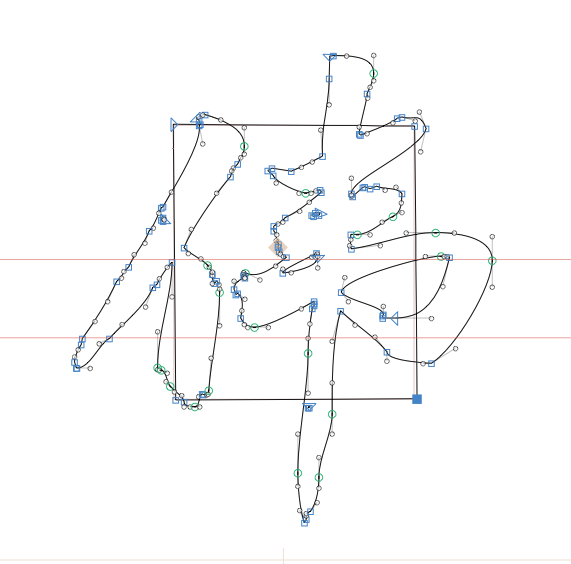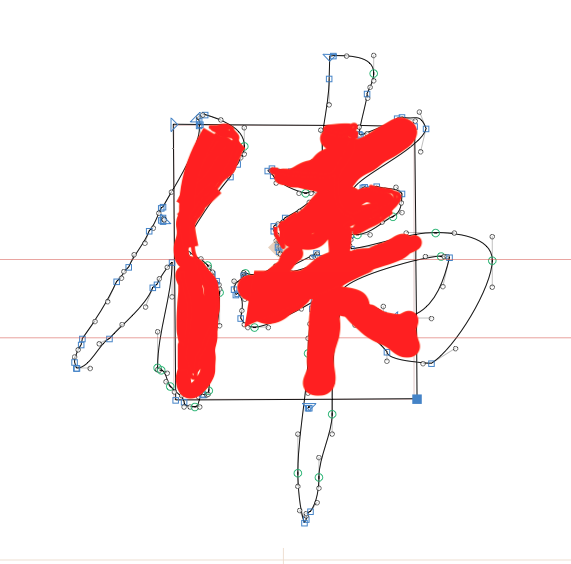# Measure the area of fonts

Hello, how to measure the area of fonts in a rectangular box area, as shown in the figure.Which area are you trying to calculate? The white space within the box?

This can get very tricky, depending on your method. For pure polygons, it’s simpler:

``````def calculate_polygon_area(coordinates):
n = len(coordinates)
if n < 3:
return 0

area = 0.0
j = n - 1

for i in range(n):
area += (coordinates[j] + coordinates[i]) * (coordinates[j] - coordinates[i])
j = i

return abs(area) / 2

# Example usage:
polygon_coordinates = [(0, 0), (4, 0), (4, 3), (2, 5), (0, 3)]
area = calculate_polygon_area(polygon_coordinates)
print("Polygon area:", area)
``````

For bezier curve segments (requires the `bezier` and numpy python packages):

``````import numpy as np

def calculate_bezier_area(bezier_segments, num_subdivisions):
total_area = 0.0
for bezier_segment in bezier_segments:
# Uniformly subdivide the bezier curve segment
t_values = np.linspace(0, 1, num=num_subdivisions)
points = bezier_segment.evaluate_multi(t_values)

# Calculate the centroid of the shape
centroid = np.mean(points, axis=0)

# Calculate the area of each triangle formed by centroid and adjacent points
for i in range(num_subdivisions - 1):
triangle_points = [centroid, points[i], points[i + 1]]
total_area += calculate_triangle_area(triangle_points)

def calculate_triangle_area(points):
x = [point for point in points]
y = [point for point in points]
return 0.5 * np.abs(np.dot(x, np.roll(y, 1)) - np.dot(y, np.roll(x, 1)))

# Example usage:
from bezier import Curve
bezier_segments = [
Curve(np.array([[0, 0], [1, 3], [3, 1], [4, 4]])),
Curve(np.array([[4, 4], [5, 2], [7, 4], [8, 1]]))
]
num_subdivisions = 100
area = calculate_bezier_area(bezier_segments, num_subdivisions)
print("Shape area:", area)
``````

The required area is the area inside the path, which is the area of the font in the rectangular box, as shown in the red part of the figureThen good luck with the second code snippet I sent.

Thank you. I’ll give it a try

The degree parameter is missing, “Curve(np.array([[0, 0], [1, 3], [3, 1], [4, 4]]),degree = 1)”. Then, report an error：Traceback (most recent call last):
File “”, line 34
File “”, line 17, in calculate_bezier_area
IndexError: index 4 is out of bounds for axis 0 with size 4

There’s `path.area()` for GSPaths.

1 Like